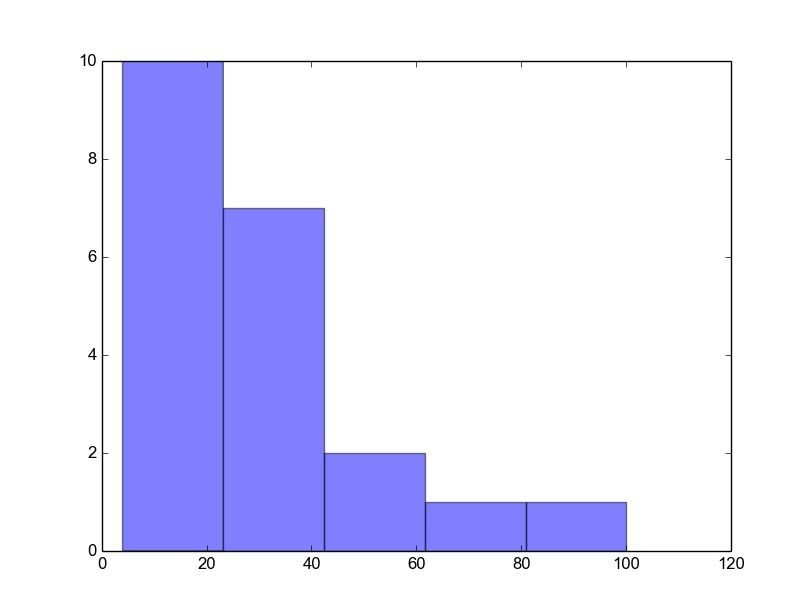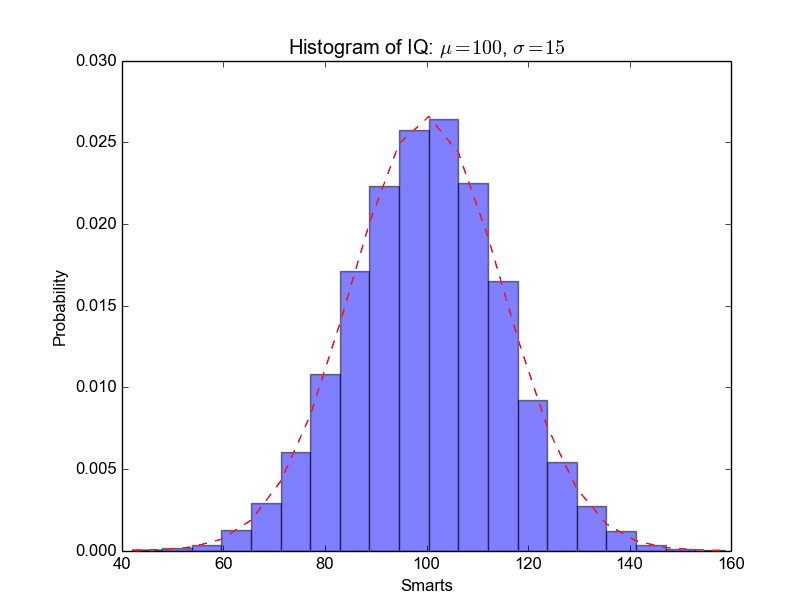# Matplotlib Histogram

Matplotlib can be used to create histograms. A histogram shows the frequency on the vertical axis and the horizontal axis is another dimension. Usually it has bins, where every bin has a minimum and maximum value. Each bin also has a frequency between x and infinite.

Related course

Matplotlib histogram example
Below we show the most minimal Matplotlib histogram:

Output:Python histogram

A complete matplotlib python histogram
Many things can be added to a histogram such as a fit line, labels and so on. The code below creates a more advanced histogram.

Output:python_histogram